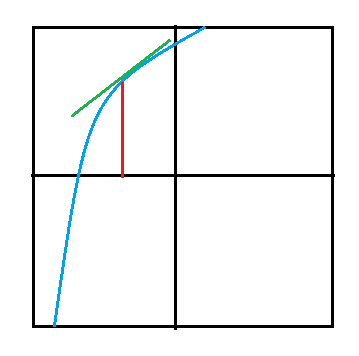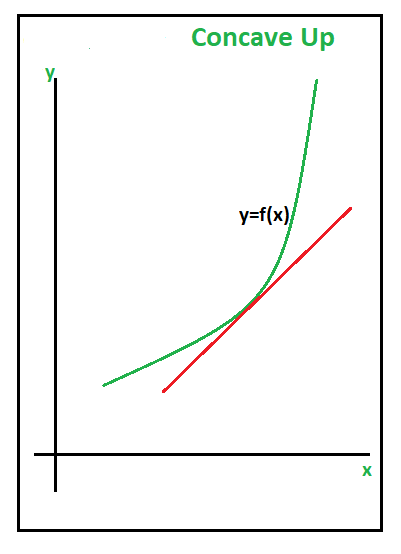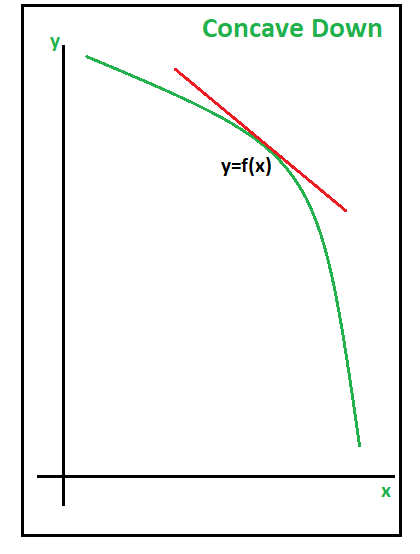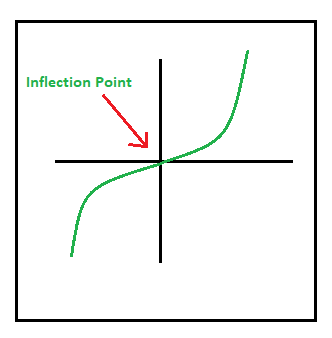Related Articles

# Second Order Derivatives in Continuity and Differentiability | Class 12 Maths

• Difficulty Level : Expert
• Last Updated : 26 Nov, 2020

The Second Order Derivative is defined as the derivative of the first derivative of the given function. The first-order derivative at a given point gives us the information about the slope of the tangent at that point or the instantaneous rate of change of a function at that point. Second-Order Derivative gives us the idea of the shape of the graph of a given function. The second derivative of a function f(x) is usually denoted as f”(x). It is also denoted by  D2y or y2 or y” if y = f(x)

Let y = f(x)

Then, dy/dx = f'(x)

If f'(x) is differentiable, we may differentiate (1) again w.r.t x. Then, the left-hand side becomes d/dx(dy/dx) which is called the second order derivative of y w.r.t x.

Example 1: Find d2y/dx2, if y = x3?

Solution:

Given that, y = x3

Then, first derivative will be
dy/dx = d/dx (x3) = 3x2

Again, we will differentiate further to find its
second derivative,

Therefore, d2y/dx2 = d/dx (dy/dx)

= d/dx (3x2)

= 6x

Note: d/dx (xn) = nxn – 1, where n is the power raised to  x.

Example 2: Find d2y/dx2, if y = Asinx + Bcosx, Where A and B are constants?

Solution:

Given that, y = Asinx + Bcosx

Then, first derivative will be

dy/dx = d/dx (Asinx + Bcosx)

= A d/dx (sinx) + B d/dx (cosx)

= A(cosx) + B(-sinx)

= Acosx – Bsinx

Again, we will differentiate further to find its second derivative,

d2y/dx2  = d/dx (dy/dx)

= d/dx (Acosx – Bsinx)

= A d/dx (cosx) – B d/dx (sinx)

= A(-sinx) – B(cosx)

= -Asinx – Bcosx

= -(Asinx + Bcosx)

= -y

Note:

d/dx (sinx) = cosx

d/dx( cosx) = -sinx

Example 3: y = logx, Find d2y/dx2?

Solution:

Given that, y = logx

Then first derivative will be,

dy/dx = d/dx (logx)

= (1 / x)

Again, we will further differentiate to find its second derivative,

d2y/dx2 = d/dx (dy/dx)

= d/dx (1 / x)     (from first derivative)

= -1 / x2

Example 4: y = ex sin5x, Find d2y/dx2?

Solution:

Given that, y = ex sin5x

Then first derivative will be,

dy/dx = d/dx (ex sin5x)

= ex d/dx (sin5x) + sin5x d/dx (ex)     (multiplication rule)

= ex (cos5x . 5) + sin5x . ex

= ex (5cos5x + sin5x)

Again, we will further differentiate to find its second derivative,

d2y/dx2 = d/dx (dy/dx)

= d/dx (ex (5cos5x + sin5x))

= ex d/dx (5cos5x + sin5x) + (5cos5x + sin5x) d/dx(ex)

= ex (d/dx (5cos5x) + d/dx (sin5x)) + (5cos5x + sin5x) d/dx (ex)

= ex(5(-sin5x)5 + 5cos5x) + (5cos5x + sin5x)(ex)

= ex(-25sin5x + 5cos5x + 5cos5x + sin5x)

= ex(10cos5x – 24sin5x)

= 2ex(5cos5x – 12sin5x)

Note: Multiplication Rule of Differentiation
d(uv) / dx = (u. dv/dx) + (v. du/dx)

### Second-Order Derivatives of a Function in Parametric Form

To calculate the second derivative of the function in the parametric form we use the chain rule twice. Hence to find the second derivative, we find the derivative with respect to t of the first derivative and then divide by the derivative of x with respect to t. Suppose that x = x(t) and y = y(t), then its Parametric form in Second Order:

First Derivative: dy/dx = (dy/dt) / (dx/dt)

Second Derivative: d2y/dx2 = d/dx (dy/dx)

= d/dt (dy/dx) / (dx/dt)

Note: It is totally wrong to write the above formula as d2y/dx2 = (d2y/dt2) / (d2x/dt2)

Example: If x = t + cost, y = sint, find the second derivative.

Solution:

Given that, x = t + cost and y = sint

First Derivative,

dy/dx = (dy/dt) / (dx/dt)

= (d/dt (sint)) / (d/dt (t + cost))

= (cost) / (1 – sint)                                                               —- (1)

Second Derivative,

d2y / dx2 = d/dx (dy/dx)

= d/dx (cost / 1 – sint)                                                     —- (from eq.(1))

= d/dt (cost / 1 – sint) / (dx/dt)                                       —- (chain rule)

= ((1 – sint) (-sint) – cost(-cost)) / (1 – sint)2 / (dx/dt)      —- (quotient rule)

= (-sint + sin2t + cos2t) / (1 – sint)2 / (1 – sint)

= (-sint + 1) / (1 – sint)3

= 1 / (1 – sint)2

Note:

1) Quotient Rule of Differentiation: dy/dx = v(du/dx) – u(dv/dx) / v2

2) Chain Rule: dy/dx = (dy/du) . (du/dx)

### Graphical Representation of Second-Order Derivatives

Graphically the first derivative represents the slope of the function at a point, and the second derivative describes how the slope changes over the independent variable in the graph.In this graph, the blue line indicates the slope, i.e. the first derivative of the given function. For example, we use the second derivative test to determine the maximum, minimum, or point of inflection. The second derivative of a given function corresponds to the curvature or concavity of the graph. If the second-order derivative value is positive, then the graph of a function is upwardly concave. If the second-order derivative value is negative, then the graph of a function is downwardly open.

Concavity of Function

Let f(x) be a differentiable function in a suitable interval. Then, the graph of f(x) can be categorized as:

Concave Up: A section of a curve is concave up if the y-value grows at a faster and faster rate moving from left to right.Concave Down: The opposite of concave up, in which the y-value decreases from left to right, is called concave down.Points of Inflection: Inflection points are points where the function changes concavity, i.e. from being “concave up” to being “concave down” or vice versa.The second derivative of a function determines the local maximum or minimum, inflection point values. These can be identified with the help of the below conditions:

• If f”(x) < 0, then the function f(x) has a local maximum at x.
• If f”(x) > 0, then the function f(x) has a local minimum at x.
• If f”(x) = 0, then it is not possible to conclude anything about the point x

Attention reader! Don’t stop learning now. Participate in the Scholorship Test for First-Step-to-DSA Course for Class 9 to 12 students.

My Personal Notes arrow_drop_up